## Sunday, July 11, 2021

### Data Preprocessing Vs. Data Wrangling

• Data Preprocessing is performed before Data Wrangling
• Data Preprocessing data is prepared exactly after receiving the data from the data source.
• In this initial transformations, Data Cleaning or any aggregation of data is performed. It is executed once.
• It is the concept that is performed before applying any iterative model and will be executed once in the project.
• Data Wrangling is performed during the iterative analysis and model building.
• This concept at the time of feature engineering.
• The conceptual view of the dataset changes as different models is applied to achieve good analytic model.

### Preparing Own Collected Dataset as Benchmark Dataset for Research

1. Must be publicly available (upload on online portal and without any permission / OPEN ACCESS)

2. The dataset must address a specific problem / instance (CLASSIFICATION / REGRESSION / CLUSTERING / ENSEMBLED / DECISION TREE)

3. The dataset should not be generic for all algorithms..... dataset should not be like an all-rounder

4. Preferably Standardized (standard deviation based, consistent variance : statistical formulations should be used)

## Tuesday, October 6, 2020

Generative Adversarial Networks (GAN) is one of the key domains of research in Advanced Machine Learning and Deep Learning Applications. It is used for text generation, forensic applications, network domain and many others

## Saturday, June 20, 2020

### Import and Match Multiple DataFrames from EXCEL using PYTHON PANDAS

Many times, there is need to concatenate and analyze multiple data frames in Python.

Suppose we have Three different Excel Sheets in which same type of attributes are there. From these excel sheets, the data can be imported to data frames and then analysis can be done.

Following are three different MS Excel Sheets of attendance of the candidates. From these sheets, we have to check whether a candidate attended all sessions or not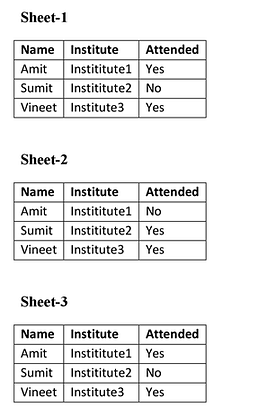`PYTHON CODE`
`import pandas as pddf1 = pd.read_excel (r'Book1.xlsx')df1 = pd.DataFrame(df1, columns= ['Name', 'Attended'])df2 = pd.read_excel (r'Book2.xlsx')df2 = pd.DataFrame(df2, columns= ['Attended'])df3 = pd.read_excel (r'Book3.xlsx')df3 = pd.DataFrame(df3, columns= ['Attended'])print('---Sheet-1---')print (df1)print('---Sheet-2---')print (df2)print('---Sheet-3---')print (df3)frames = [df1, df2, df3]result = pd.concat(frames, axis=1, sort=False)print(result)`

Output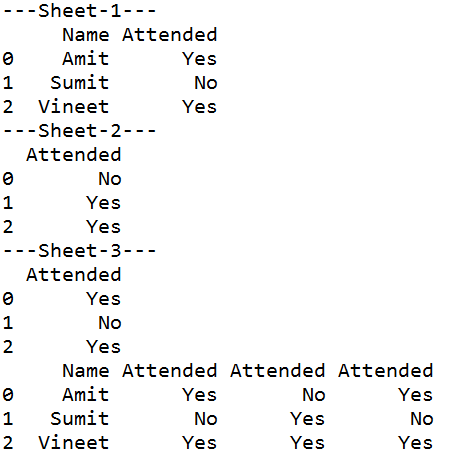## Sunday, April 19, 2020

### Python Code: Synchronization and Race-Conditions with Multi-Threading

Thread synchronization: Mechanism to ensures that two or more concurrent threads do not simultaneously execute some particular program segment known as critical section

Critical section refers to the parts of the program where the shared resource is accessed.

Issues in Synchronization

Race Condition: Occurring of a condition when two or more threads can access shared data and then try to change its value at the same time. Due to this, the values of variables may be unpredictable and vary depending on the timings of context switches of the processes.

Python Code: Without Synchronization

x = 0
def increment_global():
global x
x += 1

for _ in range(50000):
increment_global()

def main():
global x
x = 0
t1.start()
t2.start()
t1.join()
t2.join()

if __name__ == "__main__":
for i in range(5):
main()
print("x = {1} after Iteration {0}".format(i,x))

OUTPUT
x = 100000 after Iteration 0
x = 63883 after Iteration 1
x = 82552 after Iteration 2
x = 100000 after Iteration 3
x = 68994 after Iteration 4

Python Code: Locking Mechanism and Synchronization

x = 0
def increment():
global x
x += 1

for _ in range(100000):
lock.acquire()
increment()
lock.release()

global x
x = 0
t1.start()
t2.start()
t1.join()
t2.join()

if __name__ == "__main__":
for i in range(10):
print("Iteration {0}: x = {1}".format(i,x))

OUTPUT
Iteration 0: x = 200000
Iteration 1: x = 200000
Iteration 2: x = 200000
Iteration 3: x = 200000
Iteration 4: x = 200000
Iteration 5: x = 200000
Iteration 6: x = 200000
Iteration 7: x = 200000
Iteration 8: x = 200000
Iteration 9: x = 200000

### Create EXE File from Python Code

Method 1: Generating .EXE using PyInstaller

Install PyInstaller in Anaconda Prompt. Then Execute the Python code with pyInstaller as follows: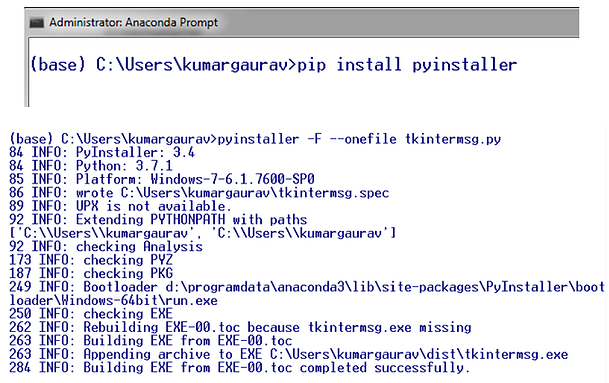Method 2: Generating .EXE using AUTO_PY_TO_EXE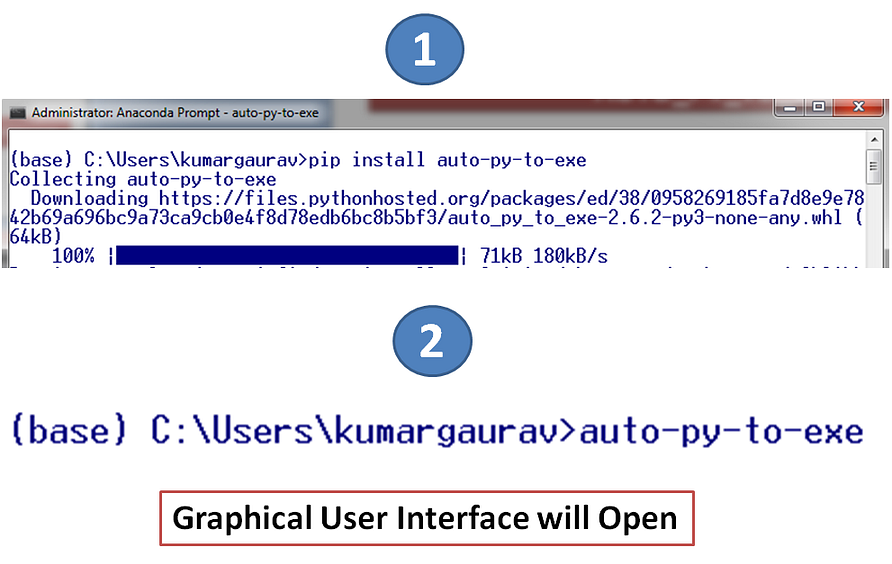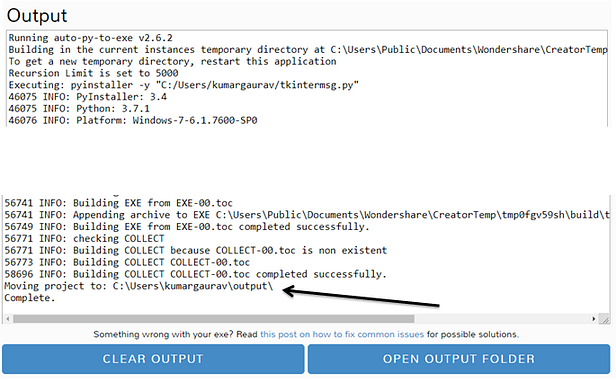### Python Code: SHA: Secured Hash Algorithm

import hashlib
print ("Available Algorithms in HASHLIB: ", end ="")
print (hashlib.algorithms_guaranteed)
print ("\r")
str = "1"
result = hashlib.sha256(str.encode())
print("SHA256 Hash: ")
print(result.hexdigest())
print ("\r")
result = hashlib.sha384(str.encode())
print("SHA384 Hash: ")
print(result.hexdigest())